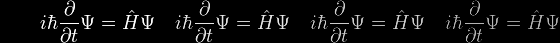# Eqn 14f Help

Most generally from the integration process, s/a = sinh(x/a)

But tanϕ = dy/dx = sinh(x/a) also, as already established,

and so tanϕ = s/a or as more usually expressed, s = a tanϕ

The eminent Victorian academic William Whewell called this kind of relationship between the curve-length and tangent at an arbitrary point on a curve, the intrinsic equation of that curve and much more general than the customary coordinate representation.

But it's curious that a crudely-simplified model of the catenary also leads to the very same intrinsic equation. Let's suppose that all the weight W of the catenary is concentrated into a single lump suspended from point C. Being themselves now weightless, the two strands AC and BC become absolutely straight lines, and the problem becomes purely trigonometrical.

We can define the geometry as

 • [Eqn (ia)] ℓ cosϕ = d • [Eqn (ib)] ℓ sinϕ = h • [Eqn (ic)] d tanϕ = h and the physics becomes • [Eqn (iia)] W = w • 2ℓ • [Eqn (iib)] W = 2T sinϕ = 2T (h/ℓ) so that • [Eqn (iic)] w • 2ℓ = 2T (h/ℓ) and T = wℓ2/h Thus we can develop the parameters T0 and a as follows • [Eqn (iiia)] T0 = T cosϕ = T (d/ℓ) = (wℓ2/h) • (d/ℓ) = wdℓ/h • [Eqn (iiib)] a = T0 /w = dℓ/h = ℓ / tanϕ and arrive at the same intrinsic equation ℓ = a tanϕ as for the true catenary!